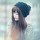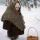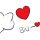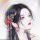##### 奸妃当道：三休蛇蝎王爷的评论 全部60条 我来说两句

•小瓶盖 2015-12-26

啥时候会更新啊？我一晚上看完了，好郁闷。。跟追电视剧一样一样的心情。。111

•maowuwu2015-12-26

看到一半，嘎然而止，太不尽兴了，云长歌还不出手41

•雨后的彩虹 2015-12-23

支持，好看，快更新，简介里应该是“苍天在上，黄土在下”还是作者故意这样写的，我不明白啊。113

•i71****2052015-12-22

很好看，女主有主见，而且不是好欺负的，作者写的很好哦，支持你！Y(^_^)Y60

•附加值ジ2015-12-22

挺好看的一本书，期待作者快点更新，我们等着40

•i37****9942015-12-22

O(∩_∩)O(^_-)-☆(^_-)-☆⊙▂⊙(^_-)-☆◑▂◑(*^．^*)◑▂◑◑▂◑(^_-)-☆(^_-)-☆^皿^^皿^;-P;-P^皿^;-P50

•柚子2015-12-21

怎么还不更新啊。等的干着急！挺好看的！赶紧更新吧60

•﹏温柔旎つ゛2015-12-20

还好吧，先留着，免费就好。呵呵(^_^)呵呵 呵呵(^_^)呵呵(^_^)(^_^)呵呵(^_^)(^_^)(^_^)(^_^)(^_^)(^_^)(^_^)(^_^)(^_^)(^_^)(^_^)(^_^)(^_^)(^_^)(^_^)(^_^)(^_^)(^_^)(^_^)(^_^)(^_^)(^_^)(^_^)(^_^)(^_^)(^_^)(^_^)(^_^)(^_^)(^_^)(^_^)(^_^)(^_^)(^_^)(^_^)(^_^)(^_^)(^_^)(^_^)(^_^)(^_^)(^_^)(^_^)(^_^)312

•i48****661 2015-12-19

应该还可以吧，先留下来，感觉还可以，免费就好，支持一下380

•月雪2015-12-18

还没看过，看简介应该是我喜欢的，支持本书！501

60 条记录 6/6 页 上一页 第一页 6